# 非Newton流体的物质点法模拟研究*

(江西理工大学 建筑与测绘工程学院；江西省环境岩土与工程灾害控制重点实验室， 江西 赣州 341000)

## 1 理 论 方 法

### 1.1 物质点法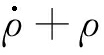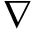·V=0;

(1)

ρa=·σ+ρb;

(2)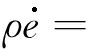·(kT)+σ∶(V),

(3)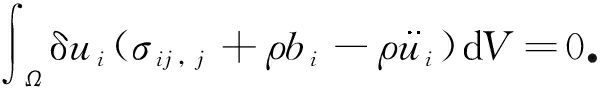(4)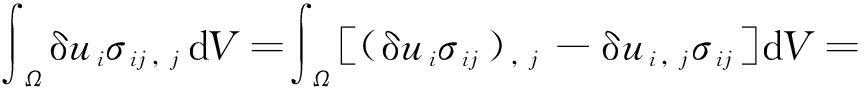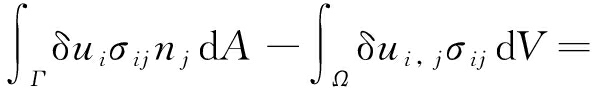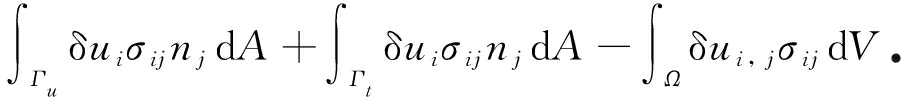(5)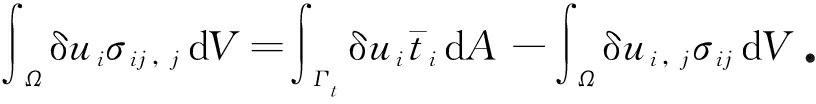(6)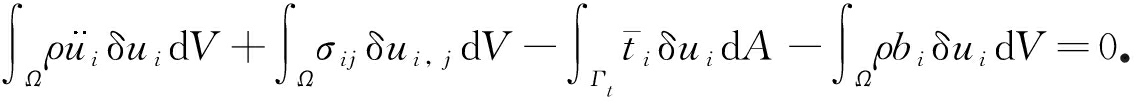(7)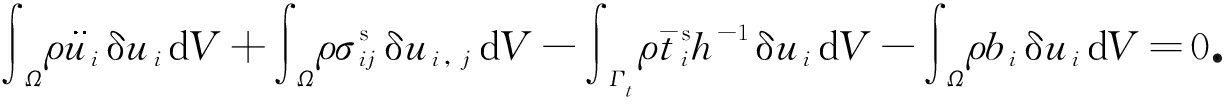(8)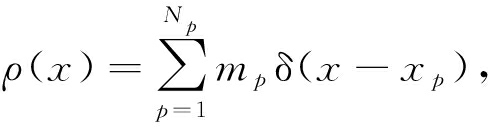(9)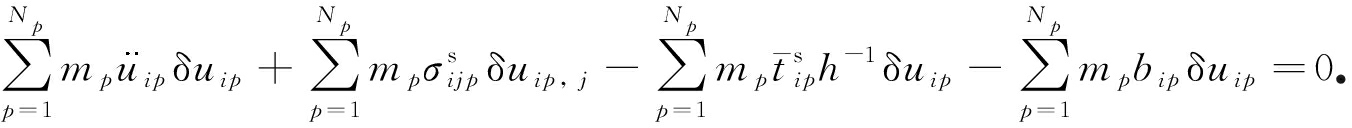(10)

uip=NIpuiI

(11)

uip, j=NIp, juiI

(12)

δuip=NIpδuiI

(13)

mI=NIpmp

(14)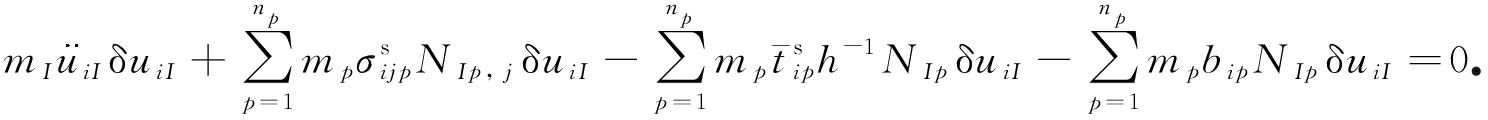(15)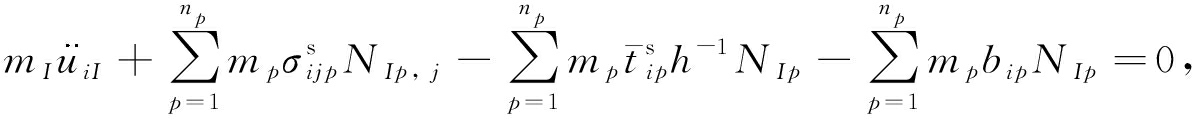(16)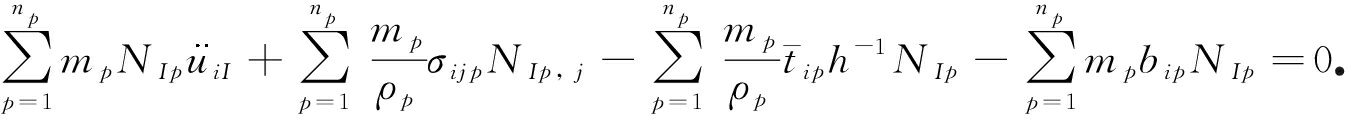(17)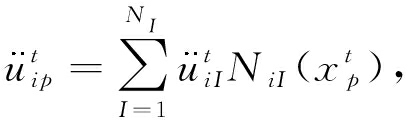(18)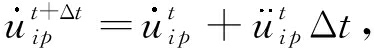(19)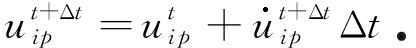(20)

### 1.2 材料模型

1.2.1 本构模型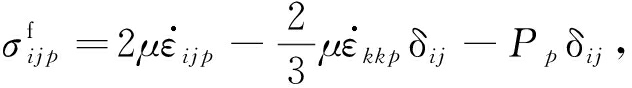(21)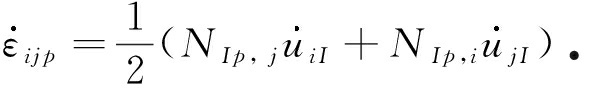(22)

1.2.2 人工状态方程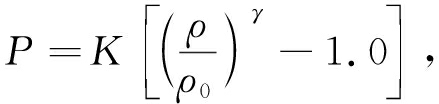(23)

1.2.3 非Newton流体模型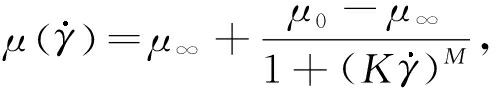(24)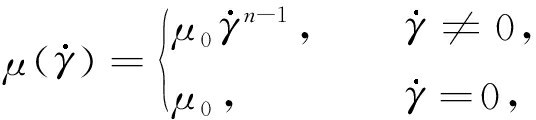(25)

## 2 计算模型及结果分析

### 2.1 Poiseuille流

Poiseuille流模型如图1所示， 静止于两固定平板中间的流体在恒定体力F作用下， 开始运动直至稳定计算模型的几何尺寸为H=1 mm和L=0.5 mm， 体积驱动力为F=8 mm/s2； 对Newton流体，其稳态最大速度为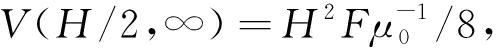即对Newton流体稳态最大速度为1 mm/s取背景网格为边长为1/20 mm的正方形，每个背景网格内布置有2×2个物质点粒子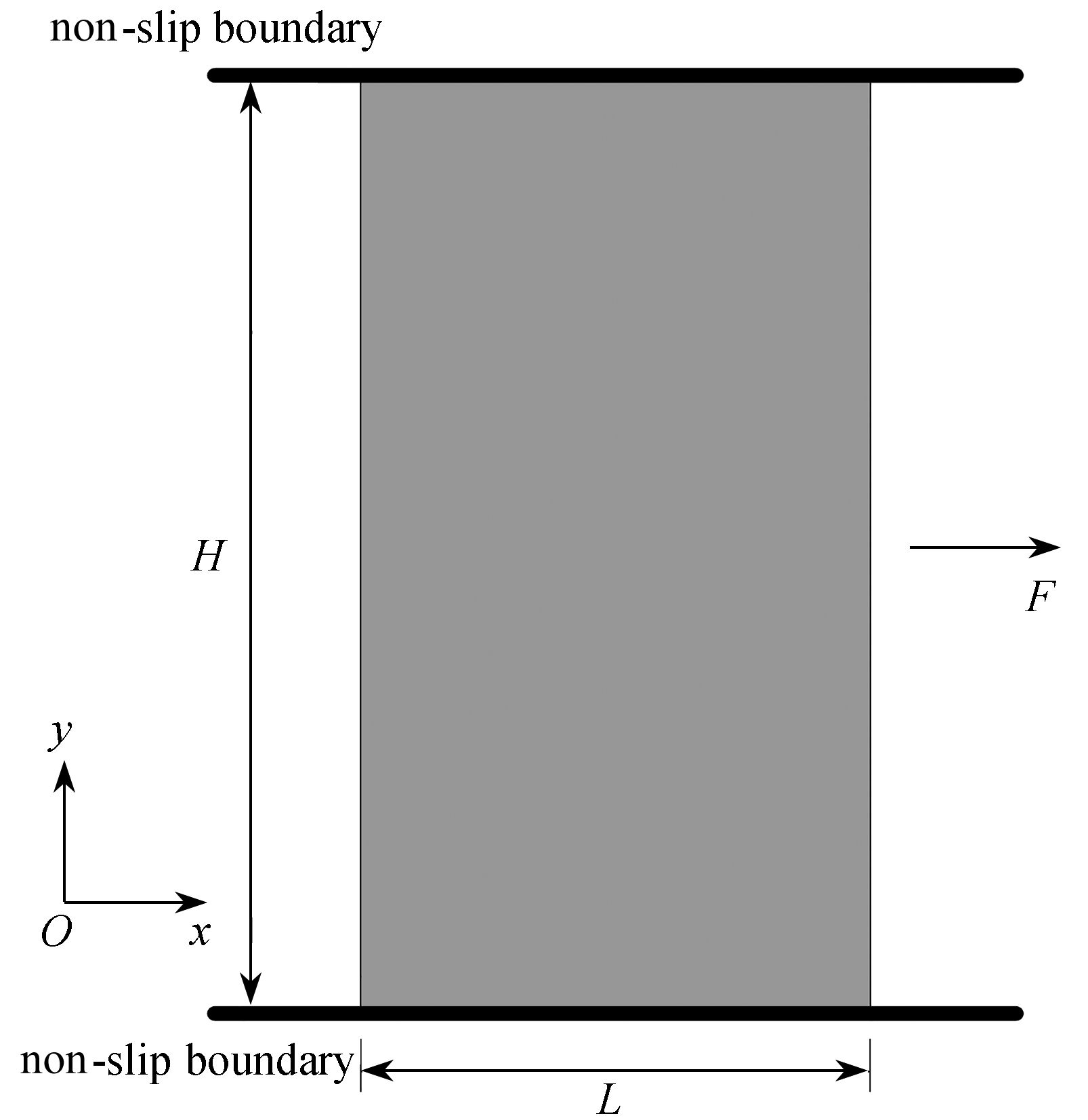Fig. 1 The model for the Poiseuille flow

Morris等给出了Newton流体Poiseuille流速度随高度和时间变化的理论解：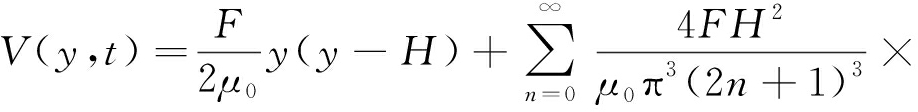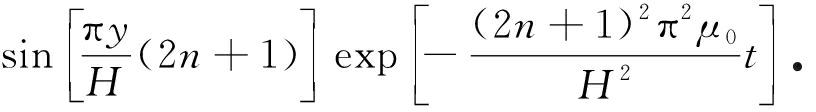(26)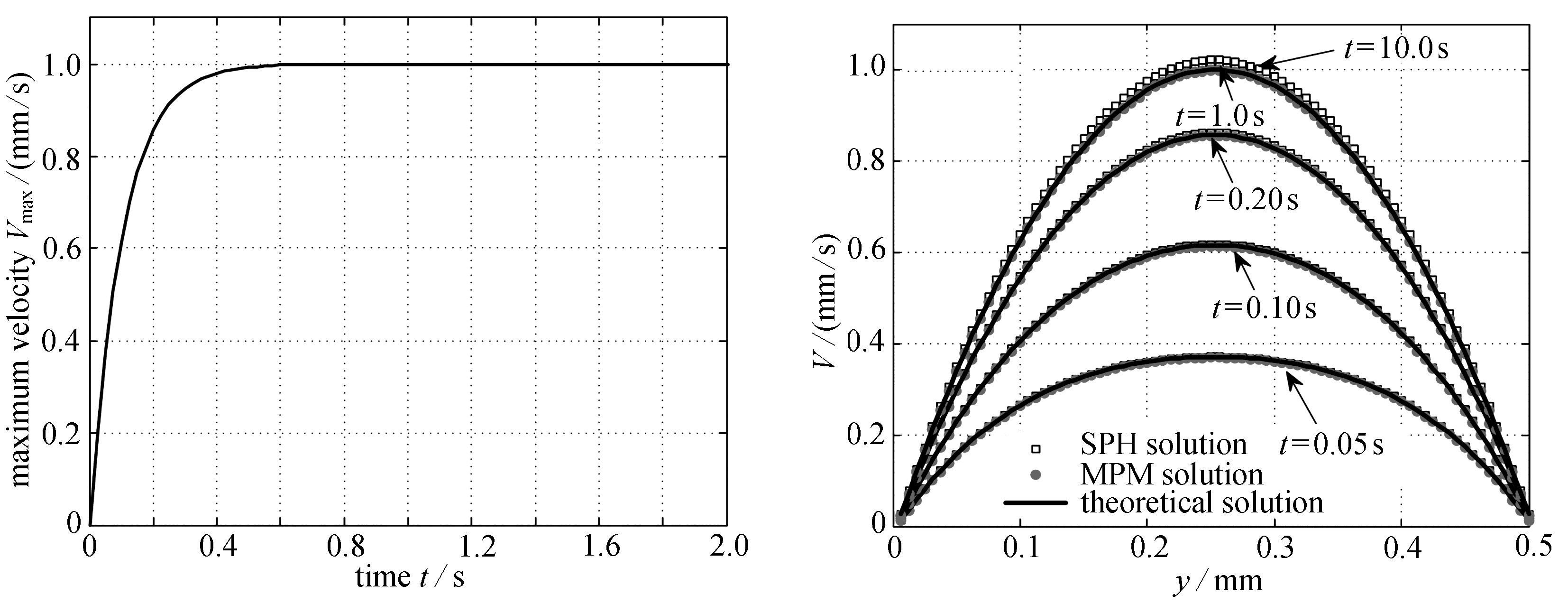(a) 最大水平速度随时间的变化规律(b) 不同时刻下物质点法和SPH法所得的
(a) The time history of the maximum velocity 速度在高度方向的分布曲线
along the x-axis (b) Comparisons of the velocity profiles between the MPM
and SPH simulations and the theoretical solutions
for the Poiseuille flow problem

Fig. 2 The MPM results of the Newtonian Poiseuille flow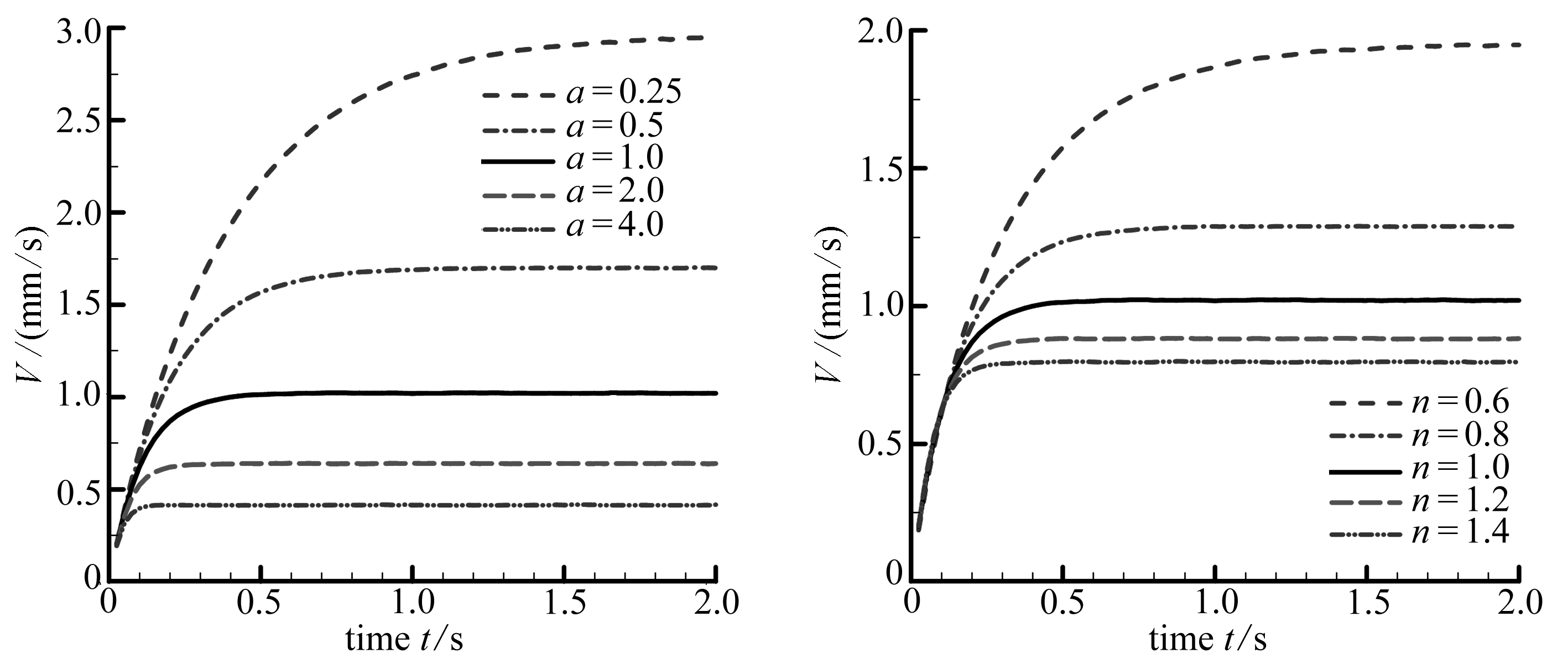(a) Cross流(b) 幂律流
(a) The cross flow (b) The power-law flow

Fig. 3 The MPM results of the non-Newtonian fluid flow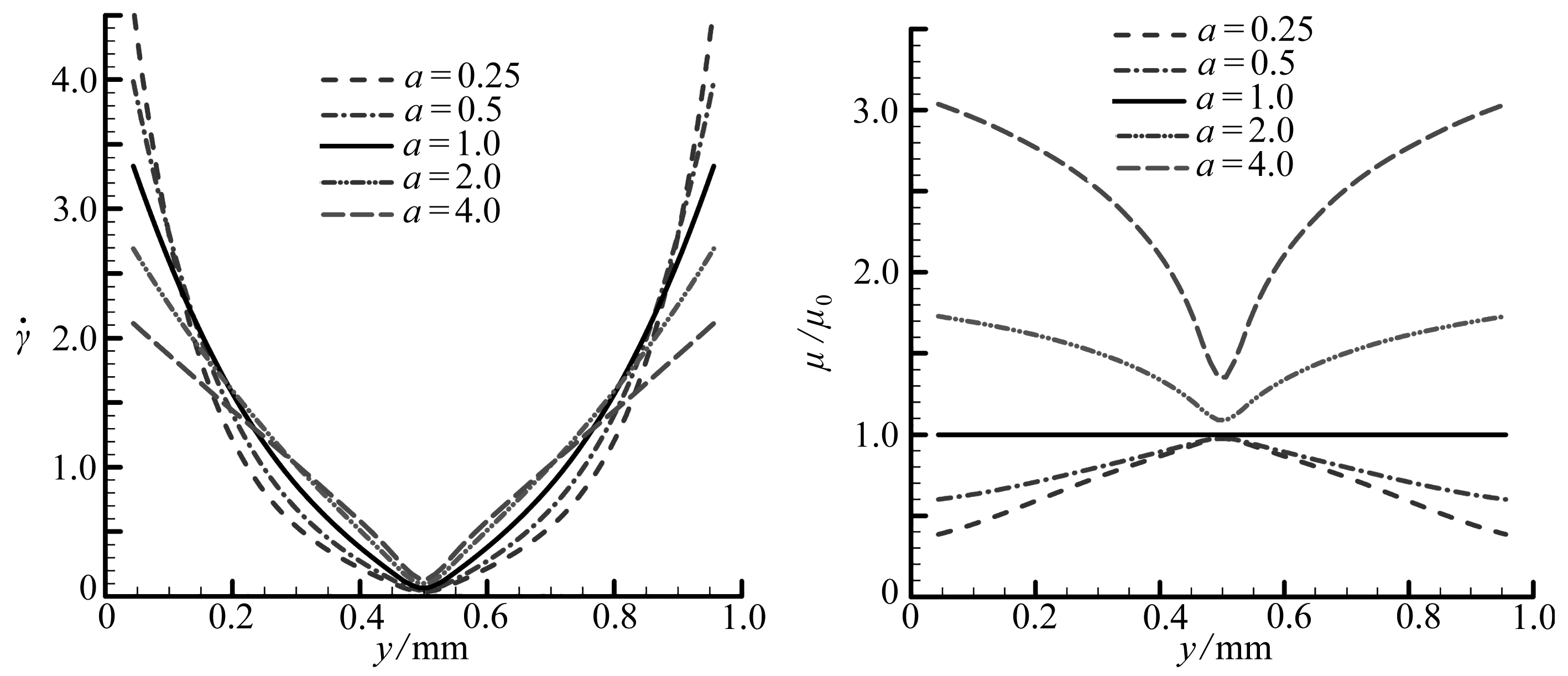(a) 平均剪切速率 (b) 平均无量纲动力黏度
(a) The average shear rates(b) The average dynamic viscosities

Fig. 4 The Poiseuille flow along the y-axis for the cross flow model at t=0.05 s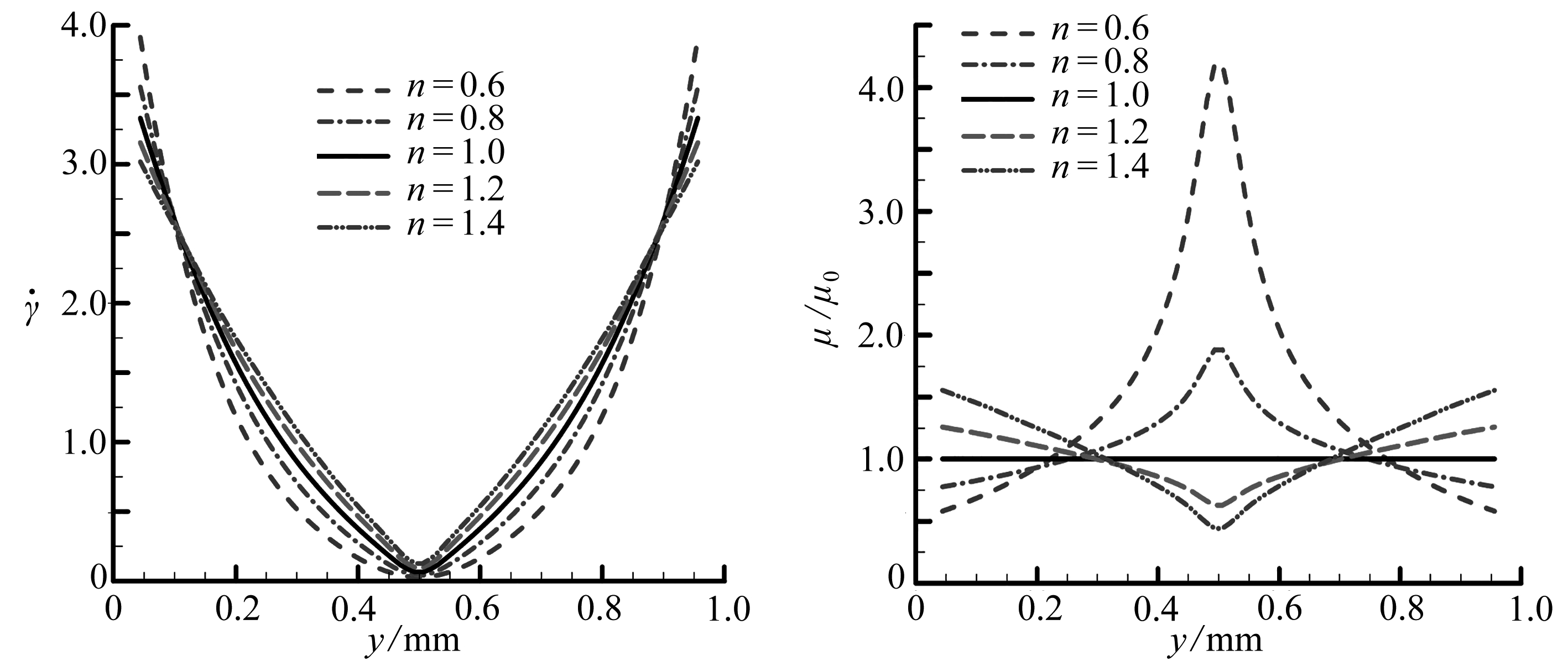(a) 平均剪切速率 (b) 平均无量纲动力黏度
(a) The average shear rates(b) The average dynamic viscosities

Fig. 5 The Poiseuille flow along y-axis for the power-law flow model at t=0.05 s

### 2.2 Couette流

Couette流模型如图6所示，下平板固定不动，上平板以恒定速度V0运动， 同时驱动初始静止于上下平板间的流体运动， 直至达到平衡计算模型的几何尺寸同样取为H=1 mm和L=0.5 mm，上平板恒定速度V0=0.1 mm/s；对Newton流体，其中间位置处的稳态速度为V(H/2,∞)=0.05 mm/s同样地，计算背景网格取边长为1/20 mm的正方形，每个背景网格内布置有2×2个物质点粒子

Morris等给出了Newton流体Couette流速度随时间和高度分布的理论解：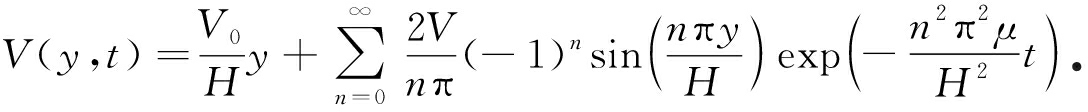(27)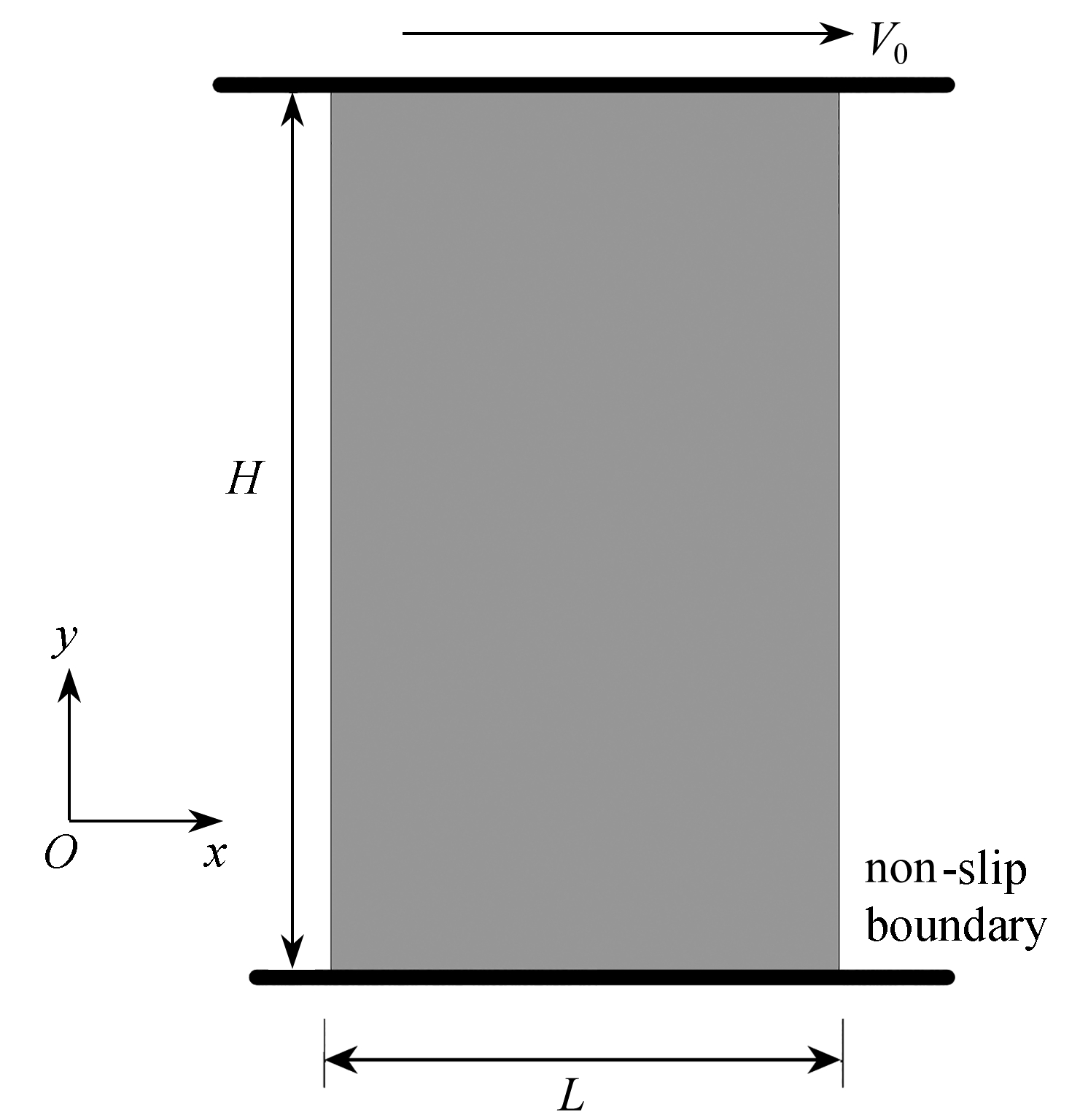Fig. 6 The model for the Couette flow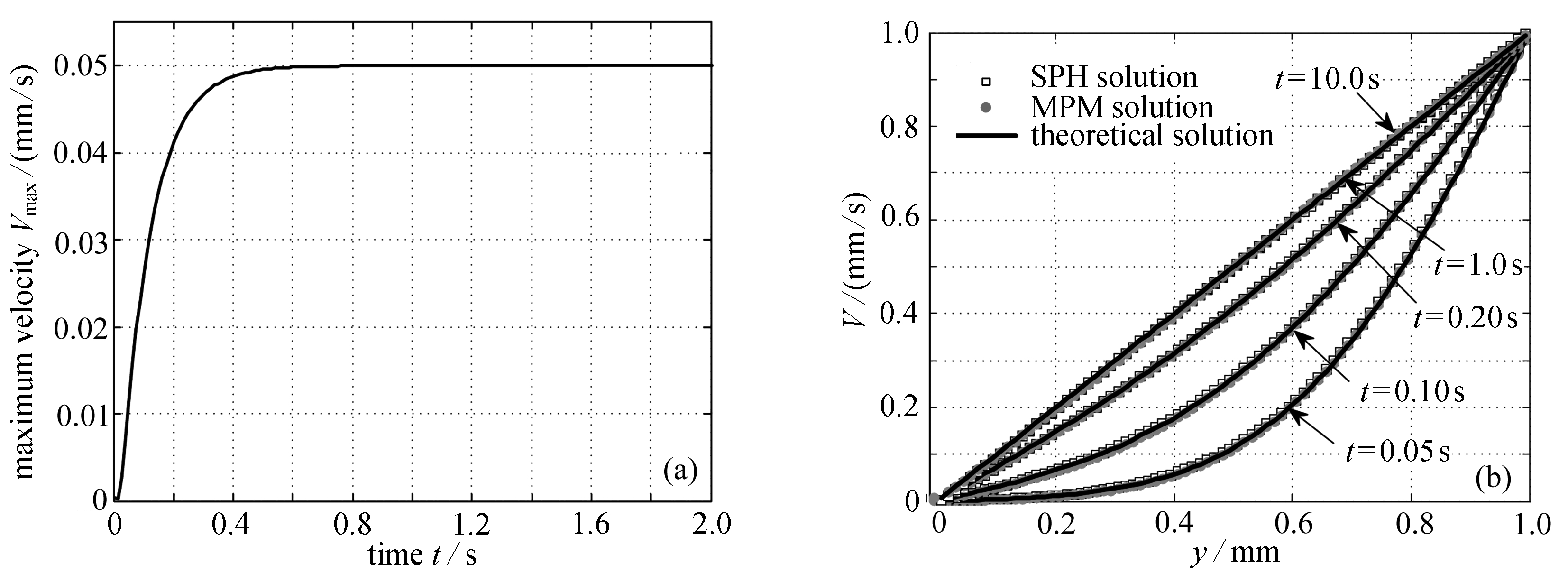Fig. 7 The MPM results of the Newtonian Couette flow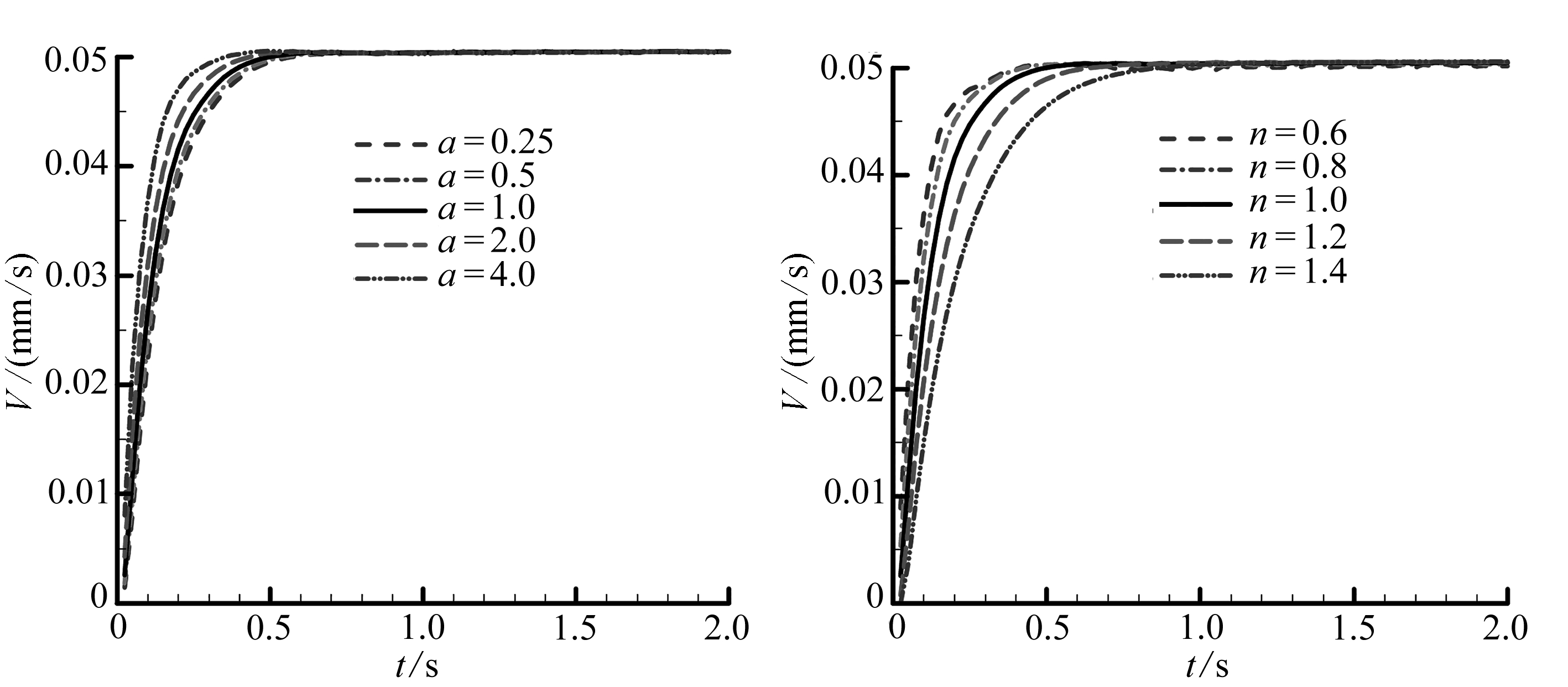(a) cross流 (b) 幂律流
(a) The cross flow (b) The power-law flow

Fig. 8 The time-histories of average velocities of the Couette flow at section y=H/2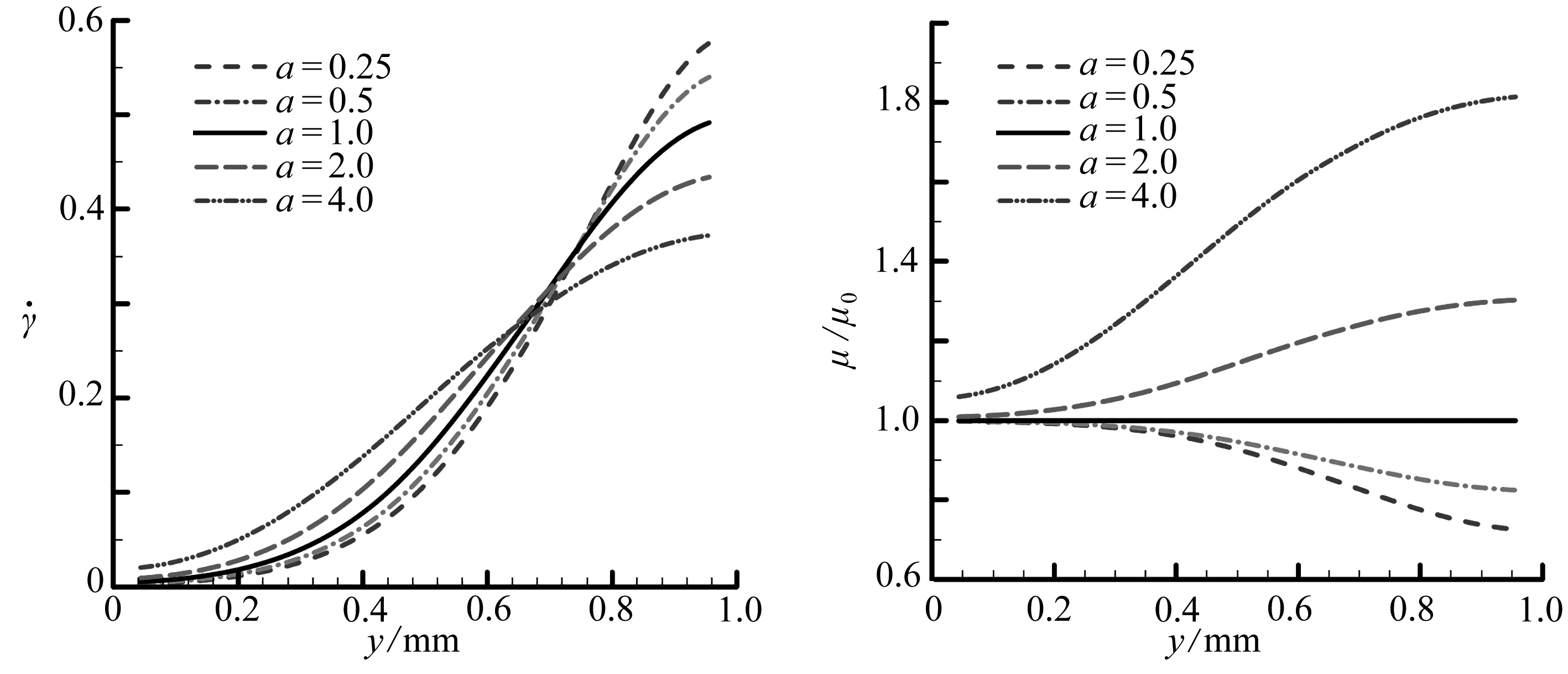(a) 平均剪切速率 (b) 平均无量纲动力黏度
(a) The average shear rates(b) The average dynamic viscosities

Fig. 9 The Couette flow along the y-axis for different cross flow models at t=0.05 s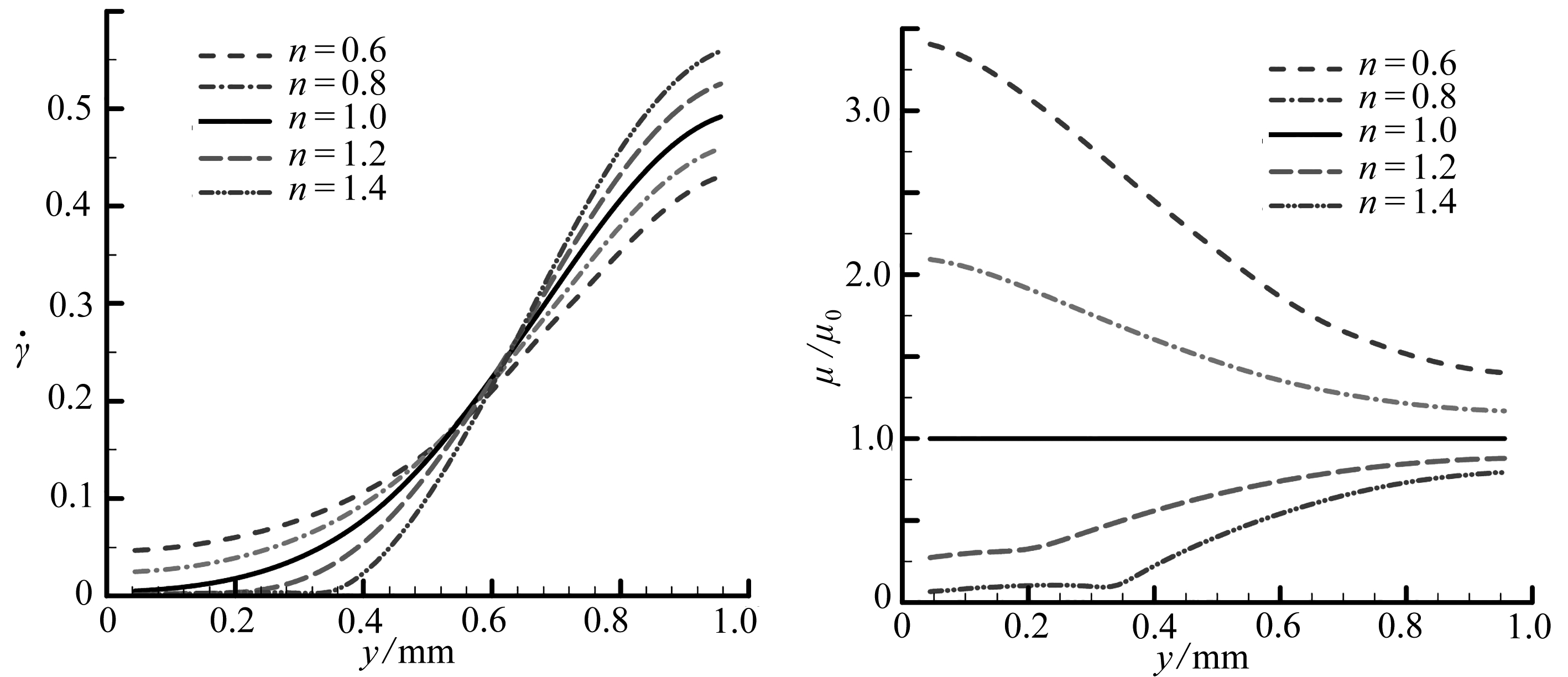(a) 平均剪切速率 (b) 平均无量纲动力黏度
(a) The average shear rates(b) The average dynamic viscosities

Fig. 10 The Couette flow along the y-axis for different power-law flow models at t=0.05 s

## 3 结 论

1) 物质点法所得Newton流体下的Poiseuille流和Couette流模拟结果与理论解吻合较好；

2) 物质点法可很好地模拟非Newton流体的流动特性，即对剪切稀化流体，随着应变速率的减小，动力黏度逐渐增大；对剪切稠化流体，随着应变速率的减小，动力黏度逐渐降低，表明了物质点法模拟非Newton流体的可行性；

3) 平板Poiseuille流的稳态速度和到达稳态时间与液体所受体力呈正比，与上下边界处的液体黏度呈反比；平板Couette流的稳态速度只与平板的移动速度有关与液体黏度无关，但其到达稳态时间与液体黏度有关

 CROCHET M J, DAVIES A R, WALTERS K. Numerical Simulation of Non-Newtonian Flow[M]. Amsterdam: Elsevier, 2012.

 BAAIJENS F P T, HULSEN M A, ANDERSON P D. The use of mixed finite element methods for viscoelastic fluid flow analysis[M]//Encyclopedia of Computational Mechanics. John Wiley & Sons, 2018: 481-498.

 张茂林, 吴清松, 李传亮, 等. 非牛顿流体的差分方法研究[J]. 西南石油学院学报, 2000, 22(1): 5-8.(ZHANG Maolin, WU Qingsong, LI Chuanliang, et al. Research on the formulation of finite difference methods for non Newtonian fluids in porous media[J]. Journal of Southwest Petroleum Institute, 2000, 22(1): 5-8.(in Chinese))

 袁世伟, 赖焕新. 幂律非牛顿流体流动的有限体积算法[J]. 华东理工大学学报(自然科学版), 2013, 39(3): 364-369. (YUAN Shiwei, LAI Huanxin. A finite volume method for calculating flows of power-law non-Newton fluids[J]. Journal of East China University of Science and Technology(Natural Science Edition), 2013, 39(3): 364-369.(in Chinese))

 GINGOLD R A, MONAGHAN J J. Smoothed particle hydrodynamics: theory and application to non-spherical stars[J]. Monthly Notices of the Royal Astronomical Society, 1977, 181(3): 375-389.

 LIU G, LIU M B. Smoothed Particle Hydrodynamics: a Meshfree Particle Method[M]. World Scientific Publishing Company, 2003.

 徐丞君, 徐胜利, 刘庆源. 修正压力梯度粒子近似SPH方法计算大密度比界面流动[J]. 应用数学和力学, 2019, 40(1): 20-35.(XU Chengjun, XU Shengli, LIU Qingyuan. Modified particle approximation to pressure gradients in the SPH algorithm for interfacial flows with high density ratios[J]. Applied Mathematics and Mechanics, 2019, 40(1): 20-35.(in Chinese))

 ZHOU G, GE W, LI J. Smoothed particles as a non-Newtonian fluid: a case study in Couette flow[J]. Chemical Engineering Science, 2010, 65(6): 2258-2262.

 FAN X, TANNER R I, ZHENG R. Smoothed particle hydrodynamics simulation of non-Newtonian moulding flow[J]. Journal of Non-Newtonian Fluid Mechanics, 2010, 165(5/6): 219-226.

 SHAMSODDINI R, SEFID M, FATEHI R. Incompressible SPH modeling and analysis of non-Newtonian power-law fluids, mixing in a microchannel with an oscillating stirrer[J]. Journal of Mechanical Science and Technology, 2016, 30(1): 307-316.

 CHAUSSONNET G, KOCH R, BAUER H, et al. Smoothed particle hydrodynamics simulation of an air-assisted atomizer operating at high pressure: influence of non-Newtonian effects[J]. Journal of Fluids Engineering, 2018, 140(6): 61301. DOI: 10.1115/1.4038753.

 SULSKY D, CHEN Z, SCHREYER H. A particle method for history-dependent materials[J]. Computer Methods in Applied Mechanics and Engineering, 1994, 118(1): 179-196.

 SULSKY D, ZHOU S, SCHREYER H. Application of a particle-in-cell method to solid mechanics[J]. Computer Physics Communications, 1995, 87(1): 236-252.

 廉艳平, 张帆, 刘岩, 等. 物质点法的理论和应用[J]. 力学进展, 2013, 43(2): 237-264.(LIAN Yanping, ZHANG Fan, LIU Yan, et al. Material point method and its applications[J]. Advances in Mechanics, 2013, 43(2): 237-264. (in Chinese))

 王宇新, 李晓杰, 王小红, 等. 炸药爆轰物质点法三维数值模拟[J]. 应用数学和力学, 2015, 36(2): 198-206.(WANG Yuxin, LI Xiaojie, WANG Xiaohong, et al. 3D simulation of explosive detonation with the material point method[J]. Applied Mathematics and Mechanics, 2015, 36(2): 198-206. (in Chinese))

 MA S, ZHANG X, QIU X M. Comparison study of MPM and SPH in modeling hypervelocity impact problems[J]. International Journal of Impact Engineering, 2009, 36(2): 272-282.

 张雄, 刘岩, 张帆, 等. 极端变形问题的物质点法研究进展[J]. 计算力学学报, 2017, 34(1): 1-16.(ZHANG Xiong, LIU Yan, ZHANG Fan, et al. Recent progress of material point method for extreme deformation problems[J]. Chinese Journal of Computational Mechanics, 2017, 34(1): 1-16.(in Chinese))

 SUN Z, LI H, GAN Y, et al. Material point method and smoothed particle hydrodynamics simulations of fluid flow problems: a comparative study[J]. Progress in Computational Fluid Dynamics, 2018, 18(1): 1-18.

 陈文芳, 蔡扶时. 非牛顿流体的一些本构方程[J]. 力学学报, 1983, 19(1): 16-26.(CHEN Wenfang, CAI Fushi. Some constitutive equations for non-Newtonian fluids[J]. Chinese Journal of Theoretical and Applied Mechanics, 1983, 19(1): 16-26.(in Chinese))

 陈文芳. 非牛顿流体力学[M]. 北京: 科学出版社, 1984.(CHEN Wenfang. The Mechanics of Non-Newtonian Fluids[M]. Beijing: Science Press, 1984.(in Chinese))

 周光坰, 严宗毅, 许世雄, 等. 流体力学(上)[M]. 北京: 高等教育出版社, 2001.(ZHOU Guangjiong, YAN Zongyi, XU Shixiong, et al. The Fluid Mechanics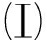[M]. Beijing: Higher Education Press, 2001.(in Chinese))

 LI J G, HAMAMOTO Y, LIU Y, et al. Sloshing impact simulation with material point method and its experimental validations[J]. Computers & Fluids, 2014, 103: 86-99.

 MORRIS J P, FOX P J, ZHU Y. Modeling low Reynolds number incompressible flows using SPH[J]. Journal of Computational Physics, 1997, 136(1): 214-226.

 MONAGHAN J J. Simulating free surface flows with SPH[J]. Journal of Computational Physics, 1994, 110(2): 399-406.

 XIANG H, CHEN B. Simulating non-Newtonian flows with the moving particle semi-implicit method with an SPH kernel[J]. Fluid Dynamics Research, 2015, 47(1): 15511. DOI: 10.1088/0169-5983/47/1/015511.

# SimulationofNon-NewtonianFluidFlowsWiththeMaterialPointMethod

ZHOU Xiaomin, SUN Zheng

(Jiangxi Provincial Key Laboratory of Environmental Geotechnique and EngineeringDisaster Control; School of Architectural and Surveying & Mapping Engineering,Jiangxi University of Science and Technology, Ganzhou, Jiangxi 341000, P.R.China)

Abstract: Simulation of the non-Newtonian fluid flow is an interesting problem for engineers. As a relatively new particle-based method, the material point method (MPM), combining the advantages of both the Lagrangian algorithm and the Eulerian algorithm, has been widely and effectively used to solve complex engineering problems. The plane Poiseuille flow and Couette flow of the shear thickening and shear thinning cross fluid and power-law fluid were studied with the artificial state equations for the MPM. The results show that, the simulation with the MPM for the Newtonian fluid is in good agreement with the theoretical solution and the MPM simulates the shear thinning and shearing thickening of the non-Newtonian fluid exactly. The results confirm the applicability of the MPM for simulation of the non-Newtonian fluid flow and expand the application field of the MPM.

Key words: material point method; non-Newtonian fluid; Poiseuille flow; Couette flow; cross flow; power-law flow

*收稿日期： 2018-12-12； 修订日期：2019-01-14

ⓒ 应用数学和力学编委会，ISSN 1000-0887

DOI: 10.21656/1000-0887.390349

Foundation item:The National Natural Science Foundation of China(11902127)